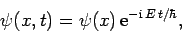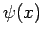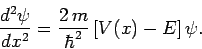Next: Infinite Potential Well Up: One-Dimensional Potentials Previous: One-Dimensional Potentials

# Introduction

In this chapter, we shall investigate the interaction of a non-relativistic particle of massand energywith various one-dimensional potentials,. Since we are searching for stationary solutions with unique energies, we can write the wavefunction in the form (see Sect. 4.12)(300)

wheresatisfies the time-independent Schrödinger equation:(301)

In general, the solution,, to the above equation must be finite, otherwise the probability densitywould become infinite (which is unphysical). Likewise, the solution must be continuous, otherwise the probability current (155) would become infinite (which is also unphysical).

Richard Fitzpatrick 2010-07-20